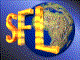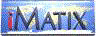| iMatix home page | << | < | > | >>SFLVersion 2.11

### compress_bits

```#include "sflcomp.h"
word
compress_bits (
byte *src,
byte *dst,
word src_size)
```

#### Synopsis

Similar to compress rle(), but optimised towards compression of sparse bitmaps. Use this when you are playing with large, sparse bitmaps. You must use expand bits () to decompress a block compressed with this function. Returns the size of the compressed data. The dst buffer should be 10% larger than the src buffer for worst cases. The src buffer must be at least src_size + 1 bytes long. It may be modified. The compressed data contains these strings:
 [00-07] Single byte containing a bit in position 0 to 7. [08-7F][data...] String of uncompressed data, 1 to 120 bytes. [82-FF] Run of 1 to 126 binary zeroes. [00-FD] Run of 127 to 380 binary zeroes. [FE][len][byte] Run of 4 to 255 identical bytes. [FF][lo][hi][byte] Run of 256 to 2^16 identical bytes. [lo][hi] Run of 381 to 2^16 binary zeroes.

#### Source Code - (sflcomp.c)

```{
word
dst_size,                       /*  Size of compressed data          */
src_scan,                       /*  Scan through source data         */
run_end,                        /*  Points to end of run of bytes    */
length = 0;                     /*  Size of the run or string        */
byte
cur_byte,                       /*  Next byte to process             */
static byte
single_bits ;              /*  Bytes with one bit set           */
static Bool
initialised = FALSE;            /*  First time flag                  */

/*  The single_bits table provides a fast lookup for bytes with          */
/*  one bit set.  The 'interesting' bytes are non-zero in the table      */
/*  where their value is the output code value (0-7) + 1.                */
if (!initialised)                   /*  First time?  Initialise          */
{
memset (single_bits, 0, 256);
single_bits    = 1;
single_bits    = 2;
single_bits    = 3;
single_bits    = 4;
single_bits   = 5;
single_bits   = 6;
single_bits   = 7;
single_bits  = 8;
}

src_scan = 0;                       /*  Start at beginning of source     */
dst_size = 0;                       /*  No output yet                    */
header   = NULL;                    /*  No open unpacked string          */
while (src_scan < src_size)
{
cur_byte = src [src_scan++];

/*- Look for 1 or more binary zeroes, and compress into a run -------*/

if (cur_byte == 0)
{
src [src_size] = 0xff;      /*  Stop with a sentinel             */
run_end = src_scan;         /*  src_scan <= src_size             */
while (src [run_end] == 0)
run_end++;

if (header)                 /*  If we have a previous unpacked   */
{                         /*    string, close it               */
*header = (byte) length + 7;
}
length = run_end - src_scan + 1;
src_scan = run_end;
if (length < 127)           /*  1-126 binary zeroes              */
dst [dst_size++] = (byte) (++length | 0x80);
else
if (length < 381)           /*  127-380 binary zeroes            */
{
dst [dst_size++] = 0x81;
dst [dst_size++] = (byte) length - 127;
}
else                        /*  381-2^16 binary zeroes           */
{
dst [dst_size++] = 0x80;
dst [dst_size++] = (byte) (length & 0xff);
dst [dst_size++] = (byte) (length >> 8);
}
}
else

/*- Next, look for bytes with 1 bit set; we encode these as 1 byte --*/

if (single_bits [cur_byte])     /*  Single bit value?                */
{
dst [dst_size++] = single_bits [cur_byte] - 1;
if (header)                 /*  If we have a previous unpacked   */
{                         /*    string, close it               */
*header = (byte) length + 7;
}
}
else

/*- Next, look for a run of 4 or more identical (non-zero) bytes ----*/

if (cur_byte == src [src_scan]
&&  cur_byte == src [src_scan + 1]
&&  cur_byte == src [src_scan + 2]
&& (src_scan < src_size - 2))
{
src [src_size] = !cur_byte; /*  Stick in a sentinel byte         */
run_end = src_scan;         /*  src_scan <= src_size             */
while (src [run_end] == cur_byte)
run_end++;

if (header)                 /*  If we have a previous unpacked   */
{                         /*    string, close it               */
*header = (byte) length + 7;
}
length = run_end - src_scan + 1;
src_scan = run_end;

if (length < 256)           /*  Short run 4-255 bytes            */
{
dst [dst_size++] = 0x81;
dst [dst_size++] = 0xFE;
dst [dst_size++] = (byte) length;
dst [dst_size++] = cur_byte;
}
else                        /*  Long run 256-2^16 bytes          */
{
dst [dst_size++] = 0x81;
dst [dst_size++] = 0xFF;
dst [dst_size++] = (byte) (length & 0xff);
dst [dst_size++] = (byte) (length >> 8);
dst [dst_size++] = cur_byte;
}
}
else

/*- Lastly, compress unpackable strings into chunks of 120 bytes ----*/

{
if (!header)                /*  Start new unpacked string if     */
{                         /*    necessary                      */
length = 0;
}
dst [dst_size++] = cur_byte;
if (++length == 120)        /*  Each string can be up to 120     */
{                         /*    bytes long (high bit cleared)  */
*header = (byte) length + 7;
 | << | < | > | >>Copyright © 1996-2000 iMatix Corporation